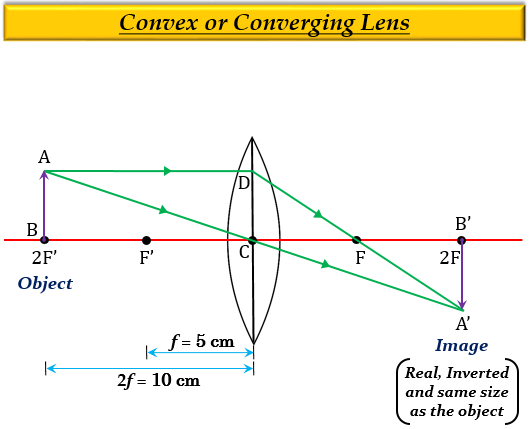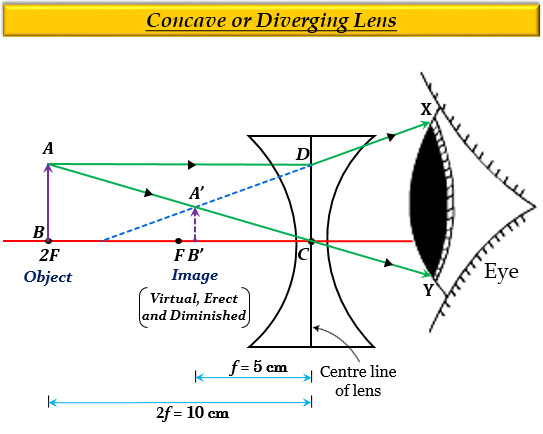# (a) An object is placed 10 cm from a lens of focal length 5 cm. Draw the ray diagrams to show the formation of image if the lens is (i) converging, and (ii) perging.(b) State one practical use each of convex mirror, concave mirror, convex lens and concave lens.

(a)(i) Here the focal length $f$ is 5 cm then $2f$ will be 10 cm $(2\times 10)$, and object is placed 10 cm from the lens, means the object is at $2F'$.

Thus, when an object is placed at $2F'$ of a converging lens, then the position of the image formed is at $2F$ behind the lens (on the right side). The nature and size of the image are real, inverted and of the same size as the object.(a)(ii) When an object is placed anywhere between optical centre $C$ and infinity (here $2F$) of a diverging lens, then the position of the image formed is on the left side of the lens, between optical centre $C$ and focus $F$. The nature and size of the image are virtual, erect, and diminished (smaller than the object).(b) One practical use of convex mirror, concave mirror, convex lens, and concave lens are as follows:

1. Convex lens $-$ Used as a magnifying glass.

2. Concave mirror $-$ Used as a shaving mirror.

3. Convex mirror $-$ Used as rear view mirror in vehicles.

4. Concave lens $-$ Used for correcting myopia.

Updated on: 10-Oct-2022

159 Views